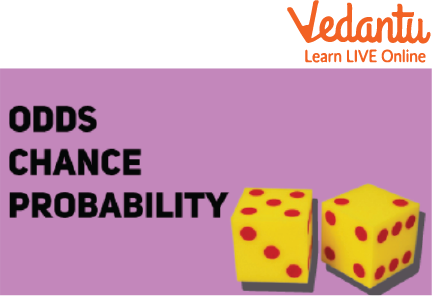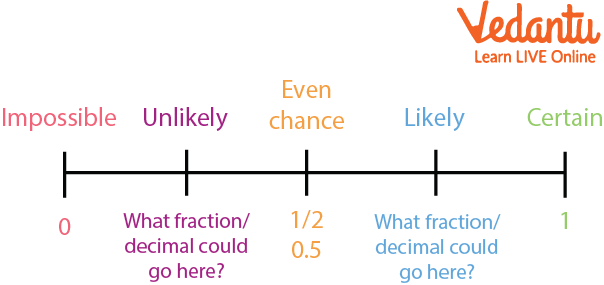Courses
Courses for Kids
Free study material
Offline Centres
More

# Odds and ProbabilityLast updated date: 03rd Dec 2023
Total views: 103.2k
Views today: 1.03k## Odds in Favour Meaning

When we do something, in mathematics we call it an event. So when an event occurred then there is some outcome or result of that event, to study that outcome or get an idea of an event we use three-term which are odds, chances, and probabilityOdds and Probability in Mathematics

## Definition of Odds

Odds:- It is a measure of the likelihood of a particular outcome and it is generally calculated by the ratio of the number of favorable outcomes to the number of unfavorable outcomes

i.e

${\rm{Odds = }}\dfrac{{{\rm{Number \; of \; favourable\; outcomes}}}}{{{\rm{Number \; of \; total \; outcomes}}}}$

## Definition of Probability and Chances

Probability:- It is the chance that something will happen, or how likely it is that an event will occur. It is calculated by the ratio of the number of favorable outcomes to the number of total outcomes

i.e

${\rm{probability = }}\dfrac{{{\rm{Number\; of \; favourable\; outcomes}}}}{{{\rm{Number \; of \; total \; outcomes}}}}$

Calculating the Probability of an event

${\rm{Probability \; of \; event = }}\dfrac{{{\rm{No}}{\rm{. of \; favourable\; outcomes}}}}{{{\rm{No}}{\rm{. of \; favourable\; outcomes + No}}{\rm{. of\; unfavourable \; outcomes}}}}$

Chances:- It is, simply, the possibility of something happening, which is not planned or controlled. Its value is the same as probability.

## Odds in Favour

Odds in favour of a particular event are given by the Number of favorable outcomes to the Number of unfavorable outcomes.

i.e Odds Formula

${\rm{odds\; in\; favour = }}\dfrac{{{\rm{No}}{\rm{. of favourable\; outcomes}}}}{{{\rm{No}}{\rm{. \;of \;unfavourable\; outcomes}}}}$

## Odds in Against

Odds against are given by Number of unfavorable outcomes to the number of favorable outcomes.

i.e

${\rm{Odds\; in\; Against = }}\dfrac{{{\rm{No}}{\rm{. of \;unfavourable \;outcomes}}}}{{{\rm{No}}{\rm{. of\; favourable\; outcomes}}}}$

## Difference between Odds and Probability

The difference between odds and probability are:

Odds of an event are the ratio of success to failure.

${\rm{Odds = }}\dfrac{{{\rm{success}}}}{{{\rm{failure}}}}$

The probability of an event is the ratio of success to the sum of success and failure.

${\rm{Probability = }}\dfrac{{{\rm{success}}}}{{{\rm{success + failure}}}}$

## Solved Examples

1. Find the odds in favor of throwing a die to get “3 dots”.

Solution:

Total number of outcomes in throwing a die = 6 (1,2,3,4,5,6)

Number of favorable outcomes = 1 (3)

Number of unfavorable outcomes = (6 - 1) = 5

Therefore, odds in favor of throwing a die to get “3 dots” is 1 : 5 or $\dfrac{1}{5}$

2. Find the odds in favor of throwing a coin to get a “tail”.

Solution:

Total number of outcomes in throwing a coin = 2 (“head”,”tail”)

Number of favorable outcomes = 1 (“tail”)

Number of unfavorable outcomes = (2- 1) = 2

Therefore, odds in favor of throwing a coin to get a “tail” is 1 : 1 or $\dfrac{1}{1}$

3. Find the odds against throwing a die to get “3 dots”.

Solution:

Total number of outcomes in throwing a die = 6

Number of favorable outcomes = 1

Number of unfavorable outcomes = (6 - 1) = 5

Therefore, odds in against of throwing a die to get “3 dots” is 5 : 1 or $\dfrac{5}{1}$

4. Find the odds against throwing a die to get “2 dots”.

Solution:

Total number of outcomes in throwing a die = 6(1,2,3,4,5,6)

Number of favorable outcomes = 1

Number of unfavorable outcomes = (6 - 1) = 5

Therefore, odds in against of throwing a die to get “3 dots” is 5 : 1 or $\dfrac{5}{1}$

5.Find the probability of getting “2 dots” in throwing a die.

Solution:

Total number of outcomes in throwing a die = 6

Number of favorable outcomes = 1

Number of unfavorable outcomes = (6 - 1) = 5

Therefore, probability of getting “2 dots” in throwing a die.

is 1: (1+5) or $\dfrac{1}{6}$

6.If odds in favor of X solving a problem are 4 to 3 and odds against Y solving the same problem are 2 to 6.

Find probability for:

(i) X solving the problem

(ii) Y solving the problem

Solution:

Given odds in favor of X solving a problem are 4 to 3.

Number of favorable outcomes = 4

Number of unfavorable outcomes = 3

(i) X solving the problem

P(X) = P(solving the problem) = 4/(4 + 3)

= $\dfrac{4}{7}$

Given odds against Y solving the problem are 2 to 6

Number of favorable outcomes = 6

Number of unfavorable outcomes = 2

(ii) Y solving the problem

P(Y) = P(solving the problem) = 6/(2 + 6)

= $\dfrac{6}{8}$

= $\dfrac{3}{4}$

In this article we learned about Odds and probability and how to calculate odds and also learnt the physical meaning of both

## FAQs on Odds and Probability

1. What is the range of probability?

The range of probability is between 0 and 12. What is meant by chance in Probability?

In real life, there are numerous instances where we must take a chance or risk. The likelihood of an event occurring based on specific circumstances may be easily anticipated. Simply put, the likelihood that a specific event will occur

3. List any two uses of Probability?

It helps in lending debt.

• Helps in commute times.

4. What is the 0 value in terms of negative or positive?

0 is neither positive nor negative. Both the terms start after or before as negative numbers or positive numbers.